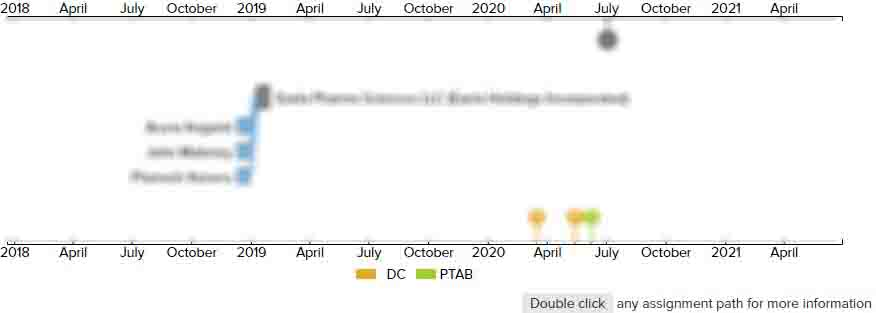# Compensation table compressing method

• US 10,652,550 B2
• Filed: 01/12/2018
• Issued: 05/12/2020
• Est. Priority Date: 12/22/2017
• Status: Active Grant
##### First Claim
Patent Images

1. A compensation table compressing method comprising steps as follows:

• acquiring multiple compensation tables of frames that are sequentially arranged;

dividing each compensation table of each frame into multiple encoding blocks, and processing each encoding block by multiple prediction modes to acquire prediction values corresponding to the prediction modes;

through a rate distortion optimizing method selecting one prediction mode with a minimum rate distortion optimizing value in each encoding block, and making subtraction between a prediction value corresponding to the prediction mode with the minimum rate distortion optimizing value in each encoding block and a value to be compressed of the encoding block to acquire a prediction error;

compression-processing the prediction error corresponding to each encoding block;

wherein;

the rate distortion optimizing value is J=D+λ

×

R, wherein D refers to a deviation between the prediction value of a corresponding encoding block and the value to be compressed, λ

refers to a weight factor, R indicates a number of bits needed for storing all information under a current prediction mode;

the prediction modes include simple inter-frame prediction, linear model prediction and intra-frame prediction;

the step of dividing each compensation table of each frame into multiple encoding blocks, and processing each encoding block by multiple prediction modes to acquire prediction values corresponding to the prediction modes, comprises;

utilizing the simple inter-frame prediction to process each encoding block to acquire first prediction values corresponding to the encoding blocks;

utilizing the linear model prediction to process each encoding block to acquire second prediction values corresponding to the encoding blocks;

utilizing the intra-frame prediction to process each encoding block to acquire third prediction values corresponding to the encoding blocks.

• ##### 1 Assignment
Timeline View
Assignment View×
×